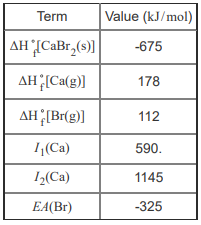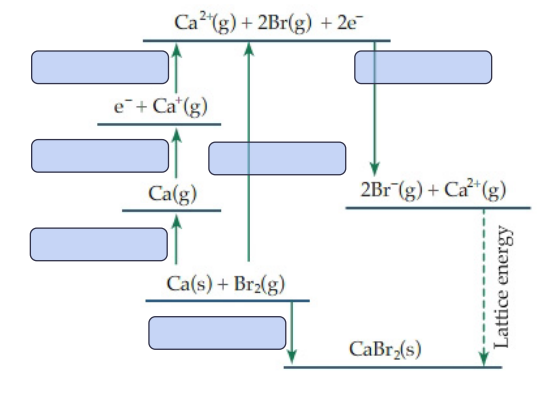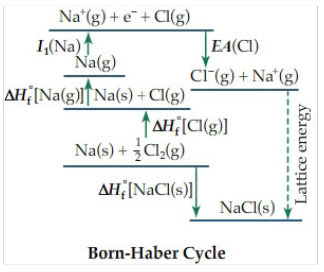# Problem: Given the following thermodynamic data, calculate the lattice energy of CaBr2(s).Express your answer to four significant figures, and include the appropriate units.By convention, the lattice energy is the energy required to form one mole of a solid ionic crystalline lattice from its gaseous ions. Since we cannot directly measure lattice energies, we generally use the Born-Haber cycle to calculate the lattice energy from other measurable quantities. For instance, the Born-Haber cycle of sodium chloride is shown below.In this cycle, the lattice energy is calculated using the following parameters. The associated reaction for each is shown in Figure 1.ΔH°f [NaCl(s)], enthalpy of formation of NaClΔH°f [Cl(g)], enthalpy of formation of gaseous ClΔH°f [Na(g)], enthalpy of formation of gaseous NaI1 (Na), first ionization energy of NaEA(Cl)], electron affinity of ClSimilar reactions and energy terms can be written to correspond to the Born-Haber cycle for the lattice energy of other ionic compounds

###### FREE Expert Solution
94% (450 ratings)View Complete Written Solution
###### Problem Details

Given the following thermodynamic data, calculate the lattice energy of CaBr2(s).Express your answer to four significant figures, and include the appropriate units.By convention, the lattice energy is the energy required to form one mole of a solid ionic crystalline lattice from its gaseous ions. Since we cannot directly measure lattice energies, we generally use the Born-Haber cycle to calculate the lattice energy from other measurable quantities. For instance, the Born-Haber cycle of sodium chloride is shown below.

In this cycle, the lattice energy is calculated using the following parameters. The associated reaction for each is shown in Figure 1.

• ΔH°f [NaCl(s)], enthalpy of formation of NaCl
• ΔH°f [Cl(g)], enthalpy of formation of gaseous Cl
• ΔH°f [Na(g)], enthalpy of formation of gaseous Na
• I(Na), first ionization energy of Na
• EA(Cl)], electron affinity of ClSimilar reactions and energy terms can be written to correspond to the Born-Haber cycle for the lattice energy of other ionic compounds